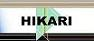| Home | Journals | Books | Paper submission | About us and our mission | News | Contact us |Site Search:

## Online Edition, Vol. 4, 2010, no. 17-20

Ch. Baba-Hamed, M. Bekkar, H. Zoubir
Translation surfaces in the three-dimensional Lorentz-Minkowski space satisfying \Delta r_i = \lambda_i r_i
Int. Journal of Math. Analysis, Vol. 4, 2010, no. 17-20, 797-808.

A. Kumar, S. K. Chaubey
A semi-symmetric non-metric connection in a generalised co-symplectic manifold
Int. Journal of Math. Analysis, Vol. 4, 2010, no. 17-20, 809-817.

I. Eryilmaz, C. Duyar
On L_w^1(G) \cap L(p,q)(G) spaces
Int. Journal of Math. Analysis, Vol. 4, 2010, no. 17-20, 819-826.

Vu Van Khuong, Mai Nam Phong
Qualitative properties for a fourth-order rational difference equation (V)
Int. Journal of Math. Analysis, Vol. 4, 2010, no. 17-20, 827-840.

Vu Van Khuong, Mai Nam Phong
A note on global behavior of solutions and positive nonoscillatory solution of rational difference equation
Int. Journal of Math. Analysis, Vol. 4, 2010, no. 17-20, 841-854.

Haidong Qu
Positive solutions of boundary value problems of nonlinear third-order differential equations
Int. Journal of Math. Analysis, Vol. 4, 2010, no. 17-20, 855-860.

B. L. Raina, H. B. Singh, K. Arunima and P. K. Raina
Sharper bounds for the zeros of polynomials using Enestrom-Kakeya theorem
Int. Journal of Math. Analysis, Vol. 4, 2010, no. 17-20, 861-872.

R. Gupta, A. K. Sharma
Operators on Lorentz-Karamata spaces
Int. Journal of Math. Analysis, Vol. 4, 2010, no. 17-20, 873-880.

rg\alpha-Homeomorphisms in topological spaces
Int. Journal of Math. Analysis, Vol. 4, 2010, no. 17-20, 881-890.

C. Boonpok
Weakly open functions on bigeneralized topological spaces
Int. Journal of Math. Analysis, Vol. 4, 2010, no. 17-20, 891-897.

M. Vali
A common fixed point theorem of semi compatible maps in fuzzy metric space
Int. Journal of Math. Analysis, Vol. 4, 2010, no. 17-20, 899-908.

S. Balasubramanian, P. Aruna Swathi Vyjayanthi
\nu D-sets and separation axioms
Int. Journal of Math. Analysis, Vol. 4, 2010, no. 17-20, 909-919.

K. V. Nagaraja, H. T. Rathod
Symmetric Gauss Legendre quadrature rules for numerical integration over an arbitrary linear tetrahedra in Euclidean three-dimensional space
Int. Journal of Math. Analysis, Vol. 4, 2010, no. 17-20, 921-928.

A. M. Ismail, M. R. MD Said, K. A. Mohd Atan, I. S. Rakhimov
Bilinear pairing computation using the extended double-base chains algorithm
Int. Journal of Math. Analysis, Vol. 4, 2010, no. 17-20, 929-941.

M. Taheri, M. Foozooni, A. Gilani
A common fixed point theorem for metrically adjoint and metrically equivalent maps
Int. Journal of Math. Analysis, Vol. 4, 2010, no. 17-20, 943-951.

Jindong Li, Li Xie
Uniqueness of meromorphic functions and differential polynomials
Int. Journal of Math. Analysis, Vol. 4, 2010, no. 17-20, 953-965.

On Osgood's criterion for classical wave equations and nonlinear shallow water wave equations
Int. Journal of Math. Analysis, Vol. 4, 2010, no. 17-20, 967-974.

S. K. Datta, S. Mandal
A result on the order and type of meromorphic functions based on CM sharing
Int. Journal of Math. Analysis, Vol. 4, 2010, no. 17-20, 975-979.

S. K. Datta, E. Jerin
Extension of some results of Datta and Jha
Int. Journal of Math. Analysis, Vol. 4, 2010, no. 17-20, 981-986.

Min Sun, Jing Li
A new family of conjugate gradient method with Armijo line search
Int. Journal of Math. Analysis, Vol. 4, 2010, no. 17-20, 987-993.

N. Ravi Shankar, V. Sireesha, K. Srinivasa Rao, N. Vani
Fuzzy critical path method based on metric distance ranking of fuzzy numbers
Int. Journal of Math. Analysis, Vol. 4, 2010, no. 17-20, 995-1006.